# A novel delta current method for transport stoichiometry estimation

## Abstract

### Background

The ion transport stoichiometry (q) of electrogenic transporters is an important determinant of their function. q can be determined by the reversal potential (Erev) if the transporter under study is the only electrogenic transport mechanism or a specific inhibitor is available. An alternative approach is to calculate delta reversal potential (ΔErev) by altering the concentrations of the transported substrates. This approach is based on the hypothesis that the contributions of other channels and transporters on the membrane to Erev are additive. However, Erev is a complicated function of the sum of different conductances rather than being additive.

### Results

We propose a new delta current (ΔI) method based on a simplified model for electrogenic secondary active transport by Heinz (Electrical Potentials in Biological Membrane Transport, 1981). ΔI is the difference between two currents obtained from altering the external concentration of a transported substrate thereby eliminating other currents without the need for a specific inhibitor. q is determined by the ratio of ΔI at two different membrane voltages (V1 and V2) where q = 2RT/(F(V2 –V1))ln(ΔI2/ΔI1) + 1. We tested this ΔI methodology in HEK-293 cells expressing the elctrogenic SLC4 sodium bicarbonate cotransporters NBCe2-C and NBCe1-A, the results were consistent with those obtained with the Erev inhibitor method. Furthermore, using computational simulations, we compared the estimates of q with the ΔErev and ΔI methods. The results showed that the ΔErev method introduces significant error when other channels or electrogenic transporters are present on the membrane and that the ΔI equation accurately calculates the stoichiometric ratio.

### Conclusions

We developed a ΔI method for estimating transport stoichiometry of electrogenic transporters based on the Heinz model. This model reduces to the conventional reversal potential method when the transporter under study is the only electrogenic transport process in the membrane. When there are other electrogenic transport pathways, ΔI method eliminates their contribution in estimating q. Computational simulations demonstrated that the ΔErev method introduces significant error when other channels or electrogenic transporters are present and that the ΔI equation accurately calculates the stoichiometric ratio. This new ΔI method can be readily extended to the analysis of other electrogenic transporters in other tissues.

## Background

Based on their electrical properties, membrane protein transporters are classified as being either electrogenic (transport a net charge) or electroneutral -. Which of these categories a given transporter belongs to is dependent on its substrate (or ion) coupling ratio; its transport stoichiometry represented by the symbol q. Electrogenic transporters are sensitive to both the electrical and chemical gradients of the ions that are being transported across a membrane. Unlike electroneutral transporters, electrogenic transporters can utilize the membrane potential of a cell or organelle membrane to drive substrates or ions against their chemical gradients. For a given electrochemical gradient, the transport stoichiometry is therefore an important independent determinant of both the magnitude and direction of substrate or ion flux through a membrane transport protein. The simplest stoichiometry for an electrogenic transporter is 1:1 as in the case of the sodium-coupled glucose transporter SGLT2 . In many instances more complex stoichiometries have been reported ,. Furthermore, certain transporters have variable stoichiometry ratios -.

The most intuitively straightforward approach for measuring the stoichiometry of a transporter is to measure the flux of each transported species either directly  or indirectly . In many instances, technical difficulties or sensitivity/specificity considerations preclude interpretable flux measurements from being acquired. Rather than measuring the actual substrate fluxes, a widely used approach is to measure the steady state current-voltage (I-V) properties of the transporter. In this approach, one determines the reversal potential (Erev), and estimates q as for example in the case of an electrogenic sodium coupled bicarbonate transporter  as follows:(1)

where intracellular concentrations of Na+ ([Na+]i) and HCO3 ([HCO3 ]i) as well as extracellular concentrations of Na+ ([Na+]o) and HCO3 ([HCO3 ]o) are known and ENBC is the reversal potential of the transporter. F, R and T are Faraday’s constant, gas constant and absolute temperature respectively. RT/F = 25.69 at 25°C .

If the electrogenic transporter under consideration is the only transport mechanism in the membrane, q estimated by solving Eq. 1 is accurate. In most cells or expression systems, there are other channels or electrogenic transporters in the membrane, reversal potential method requires the use of a specific inhibitor to differentiate the transport process of interest from other transport pathways. Subtracting the I-V curve in the presence of the inhibitor from the I-V curve without inhibitor, one obtains the Erev of the transporter-mediated current. Therefore, the relationship of Eq. 1 still holds.

Given that inhibitors are not always as specific as one would prefer, or in circumstances where a specific inhibitor is unavailable, an alternative approach has been to measure the change in zero-current membrane potential (VI=0, the voltage of the I-V curve measured at I = 0), by altering the chemical gradient(s) of the transported species -. Then ΔErev isThere are some variations of the ΔErev approach such as estimating q by determining the slope of VI=0 vs. ion or substrate concentrations . In this report, we show that ΔErev approach is correct only when the transport current under study is the only current in the membrane or in other words, currents mediated by other channels, electrogenic transporters, and leak current are negligible. When the currents mediated by other channels/transporters are not negligible, the implicit assumption underlying the ΔErev approach and its variations is that the reversal potentials due to other channels and transporters are additive to the Erev of the transporter under study, therefore they can be eliminated by subtraction. However, the assumption that Erev is additive is not valid since the effect of multiple channels/electrogenic transporters on ΔErev is a complicated function of the concentrations of ions and substrates involved, as well as the conductance and transport rate of those pathways ,.

To address these issues, we have developed a new approach named the “delta current (ΔI) method”. The utility of the ΔI approach is demonstrated using the electrogenic sodium bicarbonate cotransporters NBCe2-C and NBCe1-A ,- expressed in HEK-293 cells. In vivo, NBCe2-C is expressed in choroid plexus epithelial cells and other tissues. NBCe1-A is expressed in the mammalian kidney proximal tubule and the eye. This method has several advantages: 1) The equation does not suffer from the aforementioned errors in the ΔErev method due to other channels and functional electrogenic transporters; 2) Like the ΔErev method, the measurement protocol does not require a specific inhibitor. In addition, by computational simulations, we show the advantage of the ΔI method in calculating the stoichiometry ratio of an electrogenic transporter, and demonstrate that the ΔErev method can introduce significant errors in estimating q.

## Methods

### Expression of NBCe2-C and NBCe1-A in HEK-293 cells

The SLC4 human NBCe2-C and NBCe1-A proteins were expressed in HEK-293 cells as follows. Full-length human cDNA for each transporter was cloned into a pMSCV-IRES-EGFP (Clontech, Mountain View, CA) which expresses the transporters under a CMV promoter and also expresses EGFP as a separate protein under an internal ribosome entry site. The cDNA sequence of each of the constructs was verified by DNA sequencing. Use of human material and cell line are approved by UCLA Institutional Biosafety Committee (IBC#111.13.0-r).

### Electrophysiological recordings

Cells expressing each transporter were cultured in DMEM media with 5% FBS/5% CO2 and 37°C. The cells were transferred to 35 mm tissue culture (Bioptechs, Butler PA) inserts that were placed on the microscope stage for patch-clamp recording. The cells were continually superfused with bath solution (~2 ml/min) during the experiments. All experiments were performed in room temperature (22 ± 1°C). HEK-293 cells were whole-cell patch-clamped with the aid of fluorescent optics (Axioskop2, Carl Zeiss, Göttingen, Germany). Patch pipettes were pulled from thick wall (0.32 mm) borosilicate glass with tip size 1 - 1.5 μm (resistance: 4-6.5 MΩ). The patch pipette filling solution and bath solution components are listed in Table 1. All solutions were pH 7.4 that were confirmed with pH meter measurements in conditions throughout the studies. To ensure stable electrode potentials during whole-cell patch-clamp recordings, a micro-agar salt bridge of 2 M KCl was built in the electrode holder that formed an electrical connection between the pipette solution and the Ag/AgCl wire connected to the headstage of a patch-clamp amplifier . Intracellular signals were amplified and low pass-filtered at 400 Hz with a patch-clamp amplifier (MultiClamp 700B, Molecular Devices Co., Sunnyvale, CA). Whole cell capacitance and series resistance were determined with the auto whole-cell capacitance and series resistance compensation. The series resistance was usually compensated 80% (both prediction and correction). Junction potentials generated by different pairs of patch pipette solutions and bath solutions were determined with the junction potential calculator in software Clampex 10 (Molecular Devices Co., Sunnyvale, CA) and reported potential values were corrected for junction potentials. The inhibitor 4,4′-Diisothiocyanatostilbene-2,2′-disulfonic acid disodium salt (DIDS; SIGMA-Aldrich Co., St. Louis, MO.) was used to block NBCe2-C and NBCe1-A function.

### Data analysis

Signals from intracellular recordings were digitized at 2 KHz sampling frequency with the Digidata 1440A and software Clampex 10 (Molecular Devices Co., CA, USA). The signals were saved as data files for further analyses off-line. Data are expressed as mean ± SE. Paired t-test was used for determining statistical significance. p ≤ 0.05 was taken as the criterion for significance.

## Results

### Estimation of NBCe2-C transport stoichiometry with the conventional reversal potential method

The light microscopic image of cultured HEK-293 cells and corresponding fluorescent image of the same field is shown in Figure 1a and b respectively. Bright fluorescent cells were EGFP positive and thus were NBCe2-C expressing cells as well. We voltage-clamped EGFP positive cells at a holding voltage -60 mV and applied a series of 400 ms pulses from -95 to +45 with increment of 10 mV. The current responses to the series of pulses in pre-HCO3 (0 HCO3 ) conditions were background current due to endogenous channels in HEK-293 cells (Figure 2a left panel). We established an I-V curve of steady state current. Figure 2b shows the mean I-V curves from 8 cells. The steady state current at +45 mV was 51.8 ± 18.0 pA (mean ± SE, n = 8). Bath application of a solution containing 25 mM HCO3 (Table 1, bath solution B) induced a voltage-dependent current (Figure 2a central panel). The mean I-V curve in the presence of HCO3 is shown in Figure 2b. The steady state current at voltage +45 mV was 133.5 ± 25.5 pA (p = 0.01, paired t-test vs pre-HCO3 ). The HCO3 -induced current was obtained by subtracting the current traces in the absence of HCO3 from the current traces in its presence. Figure 2c shows the mean I-V curve of HCO3 induced current. The mean HCO3 -induced current at voltage +45 mV was 81.7 ± 23.3 pA (n = 8). The current was greatly reduced after washing with the control bath solution (Figure 2a right panel). As a separate control, we tested whether the application of HCO3 containing solution induced any current in EGFP negative cells. As shown in Figure 2d, there is no significant HCO3 -induced current detected in these cells (n = 4). These results indicate that functional NBCe2-C is expressed in EGFP labeled HEK-293 cells and that NBCe2-C transports HCO3 electrogenically.

To estimate the NBCe2-C HCO3 to Na+ transport stoichiometry q, the conventional method of measuring the reversal potential with the inhibitor DIDS was used initially. At known intracellular and extracellular concentrations of Na+ and HCO3 , q could be estimated with Eq. 1.

In this study, HEK-293 cells expressing NBCe2-C were whole-cell patch-clamped at -60 mV. VI=0 was measured in two independent experiments where [HCO3 ]i and [HCO3 ]o were equal (25 mM), therefore ENBC depended only on [Na+]i/[Na+]o. For every cell recorded, we waited at least 10 min from establishment of whole-cell patch-clamp to ensure that [Na+]i and [HCO3 ]i were equal to the concentrations of Na+ and HCO3 respectively in the patch pipette solution by diffusion before beginning I-V measurement. Current responses to a series of voltage pulses were recorded to establish I-V relationship in the absence and presence of DIDS (0.5 mM, Figure 3a). In the first experiment, using [Na+]i/[Na+]o = 40/80 mM (Patch solution d/bath solution C in Table 1), I-V curve of steady-state NBCe2-C transport current (DIDS sensitive current) was obtained by subtraction of currents in the presence of DIDS from control current (pre-DIDS). VI=0 = -22.3 ± 2.4 mV (n = 3) was obtained (Figure 3a,b and d). To show the mean and variability among cells, this VI=0 value was averaged from the VI=0 of individual sample cells. Note that this mean VI=0 value is very close to the VI=0 points where the average DIDS-sensitive I-V curve crosses the x-axis in (Figure 3b). In the second experiment using [Na+]i/[Na+]o = 25/135 mM (Patch solution c/bath solution B in Table 1), we got VI=0 = -43.9 ± 3.5 mV (n = 5, Figure 3c and d). The two VI=0 values are close to the calculated ENBC values of -17.8 and -43.3 mV (Eq. 1), respectively, assuming q = 2 (dash lines) while significantly distinct from the calculated values assuming q = 3 (dash lines, Figure 3d). The results indicate that the transport stoichiometry ratio of NBCe2-C is 2 HCO3 : 1 Na+ or (1 CO3 2−: 1 Na+) in HEK-293 cells.

### A novel delta current method for estimation of transport stoichiometry

Based on a simplified model for electrogenic secondary active transport  (as originally applied to the Na+/Ca2+ transporter), in the case of an electrogenic NBC transporter, the Na+-HCO3 flux (Jc) is shown in Eq. 2. Although we limit our evidence for the validity of our method to electrogenic NBC transporters, the approach is applicable to other electrogenic transporters.(2)

where Kc is an involved function of mobility and concentrations of free and loaded carrier  (also refer to ). zNa is the valence of Na+ and νNa is the stoichiometry of Na+. νHCO3 is the stoichiometry of HCO3 . V is the membrane potential. The total membrane current is: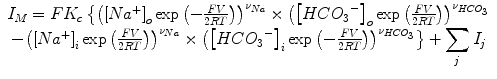(3)

Where $\sum _{j}{I}_{j}$ is the sum of all other currents mediated by various channels and electrogenic transporters including leak current on the membrane. $\sum _{j}{I}_{j}$ can be a non-linear function of V while a general assumption is that it is independent of NBC transport current.

If we change the Na+ concentration outside the cell from [Na+]o1 to [Na+]o2, the whole cell current would change from IM1 to IM2. We assume that Kc does not vary with [Na+]o within a range far from saturation. We also assume that the sum of other currents $\sum _{j}{I}_{j}$ is a function of V while the function is unchanged when [Na+]o changes (see Discussion). Therefore the delta current is(4)

$\sum _{j}{I}_{j}$ is completely eliminated. For simplicity, we take νNa = 1 and q = νHCO3Na.

Now we consider at two different voltage points V1 and V2, we have two ΔIM values, ΔIV1 and ΔIV2. We take the ratio of them,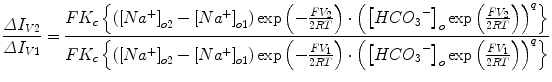(5)

ΔIV1 and ΔIV2 can be measured in electrophysiological experiments, therefore, there is only one unknown q. q can be expressed as(6)

In practical situations, to minimize the effect of the possible voltage dependence of Kc on the measurement of ΔIM and estimation of q, we take [Na+]o1 = [Na+]i and [HCO3 ]o = [HCO3 ]i, where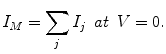Therefore, at V = 0, the delta current ΔIV1=0 is the pure NBC transport current at [Na+]o2.

q is as simple as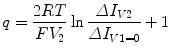(7)

In the following applications, to minimize the effects of possible Kc voltage dependence, we also take a V2 value close to 0 (e.g. ± 10 to 15 mV). Therefore the calculation involves only experimental measurements of currents close to equilibrium conditions.

### Transport stoichiometry of NBCe2-C estimated with the delta current method

Under the conditions that [Na+]i = [Na+]o = 10 mM and [HCO3 ]i = [HCO3 ]o = 25 mM (patch solution b and bath solution D in Table 1), NBCe2-C expressing HEK-293 cells were voltage-clamped at -50 mV and a series of voltage (including a pulse to 0 mV) was applied (Figure 4a, left panel). Increasing the Na+ concentration from 10 to 25 mM in the bath solution (bath solution E in Table 1) increased the voltage-dependent current (Figure 4a, central panel). Net current (ΔI) through NBCe2-C induced by changing [Na+]o was obtained by subtracting the currents in bath solution containing 10 mM Na+ from currents in 25 mM [Na+]o (Figure 4a, right panel). With this operation, according to Eq. 4, currents mediated by other channels and electrogenic transporters were eliminated if the two assumptions associated with Eq. 4 were satisfied. Figure 4b shows current-voltage (I-V) relation of steady-state current in bath solution containing 10 mM or 25 mM [Na+]o and Figure 4c shows ΔI of NBCe2-C vs. voltages. Taking ΔIv1 at V = 0 and ΔIv2 at V = 12 mV, q is calculated using Eq. 7. We obtained q = 2.0 ± 0.14 (n = 5, Figure 4d). The results suggest that the transport stoichiometry ratio of NBCe2-C is 2 HCO3 : 1 Na+ (or 1 CO3 2−: 1 Na+) in HEK-293 cells. This result is consistent with the q value obtained with the conventional reversal potential method using the inhibitor DIDS (Figure 3).

### Transport stoichiometry of NBCe1-A estimated with the delta current method

Cells expressing NBCe1-A were voltage-clamped at -50 mV, and whole-cell currents were recorded when a series of voltage pulses was applied (Figure 5a). Using the same conditions as above that [Na+]i = [Na+]o = 10 mM and [HCO3 ]i = [HCO3 ]o = 25 mM (patch solution b and bath solution D in Table 1), increasing the Na+ concentration from 10 to 25 mM in the bath solution (bath solution was switched from solution D to solution E of Table 1) increased voltage-dependent current (Figure 5a middle panel). The net current (ΔI) through NBCe1-A induced by changing [Na+]o (right panel of Figure 5a) was obtained by subtracting the current traces in the solution containing 10 mM [Na+]o from those in 25 mM [Na+]o. The current-voltage (I-V) relation of steady-state currents in bath solution containing 10 mM or 25 mM Na+ is shown in Figure 5b). Figure 5c shows ΔI of NBCe1-A vs. membrane voltages. This was the result of operation of Eq. 4 and the currents mediated by other channels and electrogenic transporters were eliminated. Taking ΔIV1 at V = 0 and ΔIV2 at V = 12 mV, we calculated q using Eq. 7 for every cell. We determined q = 1.87 ± 0.062 (n = 6, Figure 5d). The results indicate that the transport stoichiometry ratio of NBCe1-A is 2 HCO3 : 1 Na+ or 1 CO3 2−: 1 Na+ in HEK-293 cells. This estimate is consistent with our previous results using the conventional reversal potential method with DIDS .

### Computational simulation: ΔI method estimates q accurately when there are additional conductances other than electrogenic NBC transport

In native tissue or expression systems such as oocytes or HEK-293 cells, there are endogenous channels and electrogenic transporters other than the one under study. In these cases, the Δ current method is based on the assumption of additivity of membrane currents while the ΔErev method and its variations based on the assumption of additivity of reversal potentials ,,. Were the latter true, by altering the concentrations of the transported species, the contribution of other channels and electrogenic transporters could be subtracted and the relationship between delta Erev and transported species concentrations and the transport stoichiometry easily obtained based on Eq. 1. This method, although widely used, is not consistent with Goldman-Hodgkin-Katz (GHK) theory , where Erev is a logarithmic function of sum of concentrations of ions inside and outside of the membrane; i.e. not additive.

Now, suppose there is one kind of channel that is permeable to a univalent ion with valence zs and permeability of Ps on the cell membrane, in addition to an electrogenic NBC transporter. Based on Eq. 2 and the GHK current equation (with all original GHK assumptions applied ), the current would be(8)

At VI=0 of the electrogenic NBC transporter plus one channel system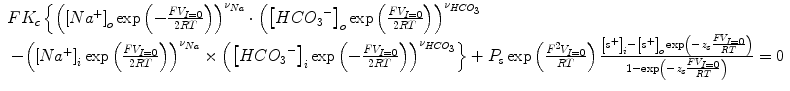(9)

We can see that even with one additional channel, this equation contains more than one unknown such as Kc, Ps and νHCO3. What we measure in the electrophysiological experiments is VI=0. VI=0 is a complicated non-additive function of ENBC. A simple expression for the relationship between stoichiometry and reversal potential is not obtained. We will see a similar situation when there is one additional electrogenic cotransporter transporting ions s1 and s2 with involved function Ka, valence Zs1 and Zs2, stoichiometry νs1 and νs2 respectively: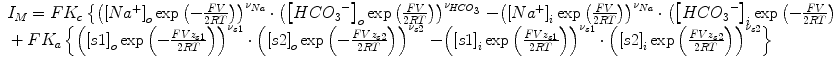(10)

Again, a simple expression for the relationship between stoichiometry and reversal potential is not obtained.

We performed a computational simulation of membrane currents and reversal potentials to show how a conductance in addition to electrogenic NBC transport affects the measurement of VI=0 and thus the estimate of q for this electrogenic NBC. Based on Eq. 2, currents were calculated with the same conditions as our whole-cell patch-clamp experiments for estimating q (delta current method above) of NBCe2-C: [HCO3 ]i = [HCO3 ]o = 25, [Na+]i =10mM. Assuming q = 2, Figure 6a shows I-V curves and VI=0s when the bath solution switched from [Na+]o =10 mM to 25 mM and the delta current (ΔI). The stoichiometry ratios estimated either with the ΔErev or ΔI methods are equivalent when there was no conductance other than the electrogenic NBC transporter (Table 2). However, if a small Cl conductance (compared to the conductance of the NBC-mediated current) was present, simulation with Eq. 8 showed that both VI=0 values at [Na+]o = 10 mM and [Na+]o = 25 mM shifted toward more negative value, but the shifts for the two conditions were different (Figure 6b). Therefore ΔErev differed from that obtained without the Cl conductance and leads to a different estimate of q = 2.17. When the Cl conductance was doubled, the estimate of q became 2.33 (Figure 6c). When we input q = 3 in the simulation, the estimate was 3 in the absence of any other conductance. After introducing either a small Cl conductance GCl or 2 x GCl (same as above), the estimate of q became 4.96 and 7.2 respectively with the ΔErev method (Figure 6d,e and f; note the insets; Table 2). However as shown in Table 2, the value of q determined using the ΔI method was unaffected by addition of a GCl on the membrane. Specifically, the ΔI-V curves in the absence, presence of small or large GCl were identical. Therefore, the currents mediated by other channels had been eliminated in the procedure and had no effect on the estimation of q.

We then simulated NBCe1-A transport in conditions similar to the proximal tubule cells in the rat kidney where the ionic concentrations (in mM) were [HCO3 ]o = 24, [HCO3 ]i = 13.4, [Na+]o = 150 and [Na+]i =17 mM . In addition to NBCe1-A, the Na+/D-glucose cotransporter SGLT2 was modeled in the simulation. SGLT2 is expressed in the apical membrane of proximal tubule cells and exhibits a transport stoichiometry of 1 Na+: 1 glucose . One positive charge moves across the membrane per transport cycle. An extracellular glucose concentration [G]o = 5 mM and intracellular [G]i = 1 mM were substituted into Eq. 10 assuming q = 2 or 3 for NBCe1-A. Table 3 shows the VI=0 values when [Na+]o = 150 and when [Na+]o was switched to 100 in the absence and presence of SGLT2. The simulation also provided estimated q values by ΔErev and ΔI methods. The stoichiometry ratios estimated either with the ΔErev or ΔI methods were equivalent when SGLT2 was absent. However, when SGLT2 was present, q was 2.55 estimated with the ΔErev method when the actual value in the simulation was 3 (Table 3). The presence of SGLT2 prevents any definitive determination as to whether the stoichiometry of NBCe1-A is q = 2 or q = 3.

These results indicate that the ΔErev method can significantly bias the estimate depending on the magnitude and electrophysiological properties (e.g. the I-V relationship) of other channels and electrogenic transporters if there are any, while the ΔI method gives a more accurate estimate of the transport stoichiometry q.

## Discussion

In this study, we have demonstrated the development and utility of a new method for estimating the transport stoichiometry of electrogenic transport proteins. With this ΔI method, one subtracts the currents due to channels and transporters other than the one under study and thereby obtains the stoichiometry of the transporter without the need for a specific inhibitor. Using this method, we showed that the transport stoichiometry of the bicarbonate cotransporter NBCe2-C expressed in HEK-293 cells is 2 HCO3 : 1 Na+ that is consistent with the results obtained using the conventional reversal potential method with the inhibitor DIDS. A transport stoichiometry ratio of 2 was also obtained for NBCe1-A with the ΔI method that is consistent with the data obtained previously using the conventional reversal potential method with DIDS . In addition, we demonstrated that, with computational simulation, the estimation of q obtained using the new ΔI method was equivalent to that obtained with the conventional ΔErev methods when an electrogenic NBC transporter was the only transport mechanism in the cell membrane. However, if a chloride channel or a glucose cotransporter SGLT2 was present in the membrane, our simulations showed that the ΔErev method significantly biased the estimate of the transport stoichiometry q, while the ΔI method gave accurate results.

The method proposed in this study is based on Eq. 2 from Heinz  that describes the functional relationship between flux of a transporter and the concentrations of transport ions/substrates and the membrane voltage . Unlike the GHK formulation that assumes independence of ion movement across the membrane  and does not involve the concept of stoichiometry, Eq. 2 explicitly expresses coupling of Na+ and HCO3 (both are voltage dependent) as a product and the stoichiometry as a power of the concentrations and voltage. Linearity of the current and voltage relation is not a presumption for Eq. 2 nor is it for the GHK equations ,. Non-linearity of the I-V curves results from: 1) the GHK equation is based on solubility-diffusion theory. In GHK current equation, the current is an exponential function of the voltage. Similarly Eq. 2 shows that flux is an exponential function of voltage; 2) transport mechanisms of membrane channels or transporters represented by the permeability term Ps in GHK equations and Kc in Eq. 2 may be voltage dependent. With the conventional Erev method, if the transporter under study is the only electrogenic pathway, this non-linearity would not be a problem since the current is 0 and at this point, the voltage is the reversal potential under the conditions of the experimental substrate concentrations. However, if there are other channels or electrogenic transporters in the membrane and if a specific inhibitor is not available, VI=0 that can be measured is not the reversal potential for the transporter under study, but rather is the voltage at a point on the I-V curve where the net result of the transporter current under study and currents mediated by other transporters and channels is 0. The alternative ΔErev method is problematic in that the assumption of reversal potential additivity is inconsistent with non-linearity property of GHK equations and Eq. 2. This is solved by employing the ΔI method where the contribution of other channels or transporters can be eliminated without the assumption of Ever additivity.

If we assume that an electrogenic NBC transporter has a fixed transport stoichiometry, if the only ions that cross the cell membrane are Na+ and HCO3 , from Eq. 2 we have(11)

When I = 0, we have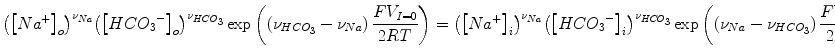(12)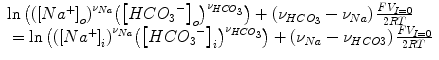(13)

Therefore,(14)

This is essentially Eq. 1 if we take νNa = 1 and q = νHCO3Na. Starting from here, the widely used delta reversal potential method to estimate stoichiometry ,, can be easily derived:

When we change Na+ concentration in the bath solution from [Na+]o1 to [Na+]o2, we have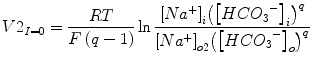(15)

Then, delta reversal potential ΔErev would be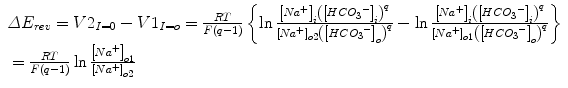(16)

From the above operations, we can see that reversal potential method, the ΔErev method and the ΔI method to estimate transport stoichiometry all have the same theoretical foundation (such as Eq. 2 and same assumptions). Moreover, they are equivalent if the electrogenic transporter under investigation is the only conductive process in the membrane.

However, if there are endogenous channels and electrogenic transporters other than the one under study, the relationship of ion activities and transport stoichiometry and reversal potential becomes very complicated as we can see in Eq. 8, Eq. 9 and Eq. 10. Therefore, a method to eliminate the confounding effects of additional transporters and channels on reversal potentials by simple subtraction of VI=0 is not valid. Our simulation results also indicate that the commonly used ΔErev method in this instance would not be accurate. The error increases as the currents mediated by other transporters and channels increase (Table 2) relative to the transporter under investigation.

Transport parameters of an electrogenic secondary active transport like Kc are affected by many factors. How a given transport process responds theoretically to an electro-chemical gradient depends on the type of the transport kinetic models utilized, e.g. “affinity model”, “velocity model” or “mixed model” as described by Heinz , and whether the loaded or the unloaded carrier bears an electrical charge. Heinz  originally introduced equation 2 and referred to Kc as a function of mobility and concentrations of the free and loaded carrier, respectively, and hence may vary with the degree of saturation. In our approach, we made two assumptions that are implicitly shared with the ΔErev method: 1) Kc is constant in certain voltage range and does not vary when the concentration of the substrate of choice in the study ([Na+]o in this study) changes; 2) the sum of currents $\sum _{j}{I}_{j}$ mediated by other channels and transporters in the membrane as a function of V does not change when the substrate concentration is altered ,,. Based on these two assumptions the two methods offer benefits such as experimentally straightforward as changing the concentrations of a substrate without the need for specific blockers and share similar limitations. The difference between ΔI and ΔErev method in terms of assumption 2 is that with the ΔI method, $\sum _{j}{I}_{j}$ can be completely eliminated (Eq. 4) if it does not change when the substrate ([Na+]o in this study) is altered. On the contrary, with the ΔErev method, as long as $\sum _{j}{I}_{j}$ is not negligible, the confounding effects of $\sum _{j}{I}_{j}$ on VI=0 can not be eliminated and biases the estimation of q as shown in Figure 6 and Table 2 and Table 3, even if it does not change when the substrate concentration varies.

In practice, ways to circumvent the limitations due to the above assumptions include: 1) using a smaller concentration change of the substrate, as long as it induces a significant delta current; 2) changing the concentrations of a particular substrate with less possibility of involving other electrogenic transporters. For example, in the case of electrogenic Na+-coupled glucose or amino acid transporters, one would choose to change either glucose or amino acids respectively rather than Na+.

In this study, we changed [Na+]o from 10 to 25 mM because: 1) HCO3 partakes in a volatile buffer system that involves pCO2 to keep the pH constant. pH would be stable when [HCO3 ]o is unaltered; 2) switching [Na+]o from 10 to 25 mM would induce a significant delta current  and 3) at these relatively low concentrations, the possibility of transport saturation would be small, therefore variation of Kc in Eq. 2 and Eq. 3 would be minimized. We assigned V1 = 0 in the above application, therefore in the conditions of ${\left[N{a}^{+}\right]}_{i}={\left[N{a}^{+}\right]}_{o}\mathit{and}\phantom{\rule{0.25em}{0ex}}{\left[HC{{O}_{3}}^{-}\right]}_{i}={\left[HC{{O}_{3}}^{-}\right]}_{o},{I}_{M}=\sum _{j}{I}_{j}$ is well defined and it is not close to 0. In addition, we assigned a V2 that is not far from 0 (+12 mV in this study), thus possible variation of Kc under extreme voltages can be minimized.

More detailed kinetic descriptions of the transport rate in order to characterize the entire I-V relationship rely on a detailed understanding of the molecular transport steps -. This is not necessary for the purposes of our formulation, because we implicitly analyze the portion of the I-V relationship that is close to the Erev i.e., V1 = 0 when [Na+]i = [Na+]o and [HCO3 ]i = [HCO3 ]o.

The accuracy of stoichiometry estimation using whole-cell patch-clamp recordings also depends on the accuracy of whole-cell current measurement and the voltages applied to the cell membrane from the patch-clamp amplifier. The drift of the junction potential between the patch pipette solution and the Ag/AgCl coated wire that connects to the headstage of the amplifier is a major source of unstable current recording especially when the Cl concentration in the pipette is low . We used a micro-agar salt bridge of 2 M KCl in the patch pipette that minimized the junction potential drift and therefore stabilized the whole-cell current measurements .

## Conclusions

We developed a new delta current (ΔI) method for estimating transport stoichiometry of electrogenic transporters based on a simplified model for electrogenic secondary active transport by Heinz (1981). We showed that this model reduces to the conventional reversal potential method when the transporter under study is the only electrogenic transport on the membrane. When there are other electrogenic transport processes such as ion channels or transporters, the ΔI method eliminates their contribution in estimation of q. We tested this new ΔI methodology in HEK-293 cells expressing the electrogenic SLC4 sodium bicarbonate cotransporters NBCe2-C and NBCe1-A, as well as using computational simulations. Our simulations demonstrated that the ΔErev method introduces significant error when other channels or electrogenic transporters are present on the membrane with a significant conductance relative to the transporter under study, and that the ΔI equation accurately calculates the stoichiometric ratio. Our new ΔI method can be readily extended to the analysis of other electrogenic transporters.

## Abbreviations

CMV:

Cytomegalovirus

DIDS:

4,4′-Diisothiocyanatostilbene-2,2′-disulfonic acid

EGFP:

Enhanced Green Fluorescent Protein

Eq:

Equation

Erev :

Reversal potential

F:

GHK:

Goldman-Hodgkin-Katz

HEPES:

4-(2-Hydroxyethyl)-1-piperazineethanesulfonic acid

I-V:

Current-voltage

NBC:

Sodium bicarbonate cotransporter

R:

Gas constant

SGLT2:

Sodium-coupled glucose transporter 2

T:

Absolute temperature

ΔErev :

Delta reversal potential

ΔI:

Delta current

## References

1. Kurtz I, Petrasek D, Tatishchev S: Molecular mechanisms of electrogenic sodium bicarbonate cotransport: structural and equilibrium thermodynamic considerations. J Membr Biol. 2004, 197 (2): 77-90. 10.1007/s00232-003-0643-x.

2. Dong H, Dunn J, Lytton J: Stoichiometry of the Cardiac Na +/Ca 2+exchanger NCX1.1 measured in transfected HEK cells. Biophys J 2002, 82(4):1943–1952.,

3. Bacconi A, Virkki LV, Biber J, Murer H, Forster IC: Renouncing electroneutrality is not free of charge: switching on electrogenicity in a Na +-coupled phosphate cotransporter. Proc Natl Acad Sci U S A 2005, 102(35):12606–12611.,

4. Wright EM, Turk E: The sodium/glucose cotransport family SLC5. Pflugers Arch. 2004, 447 (5): 510-518. 10.1007/s00424-003-1202-0.

5. Chen NH, Reith ME, Quick MW: Synaptic uptake and beyond: the sodium- and chloride-dependent neurotransmitter transporter family SLC6. Pflugers Arch. 2004, 447 (5): 519-531. 10.1007/s00424-003-1064-5.

6. Coady MJ, Chen XZ, Lapointe JY: rBAT is an amino acid exchanger with variable stoichiometry. J Membr Biol. 1996, 149 (1): 1-8. 10.1007/s002329900001.

7. Alonso GL, Gonzalez DA, Takara D, Ostuni MA, Sanchez GA: Kinetic analysis of a model of the sarcoplasmic reticulum Ca-ATPase, with variable stoichiometry, which enhances the amount and the rate of Ca transport. J Theor Biol. 2001, 208 (3): 251-260. 10.1006/jtbi.2000.2185.

8. Sacher A, Cohen A, Nelson N: Properties of the mammalian and yeast metal-ion transporters DCT1 and Smf1p expressed in Xenopus laevis oocytes. J Exp Biol. 2001, 204 (Pt 6): 1053-1061.

9. Gross E, Kurtz I: Structural determinants and significance of regulation of electrogenic Na +-HCO 3 cotransporter stoichiometry. Am J Physiol Renal Physiol 2002, 283(5):F876–F887.,

10. Iwamoto H, Blakely RD, De Felice LJ: Na +, Cl , and pH dependence of the human choline transporter (hCHT) in Xenopus oocytes: the proton inactivation hypothesis of hCHT in synaptic vesicles. J Neurosci 2006, 26(39):9851–9859.,

11. Ravera S, Virkki LV, Murer H, Forster IC: Deciphering PiT transport kinetics and substrate specificity using electrophysiology and flux measurements. Am J Physiol. 2007, 293 (2): C606-C620. 10.1152/ajpcell.00064.2007.

12. Coady MJ, Wallendorff B, Bourgeois F, Charron F, Lapointe JY: Establishing a definitive stoichiometry for the Na +/monocarboxylate cotransporter SMCT1. Biophys J 2007, 93(7):2325–2331.,

13. Hille B: Ion Channels of Excitable Membranes. 2001, Sinauer Associates, Sunderland, Massachusetts

14. Sassani P, Pushkin A, Gross E, Gomer A, Abuladze N, Dukkipati R, Carpenito G, Kurtz I: Functional characterization of NBC4: a new electrogenic sodium-bicarbonate cotransporter. Am J Physiol. 2002, 282 (2): C408-C416. 10.1152/ajpcell.00409.2001.

15. Yamaguchi S, Ishikawa T: Electrophysiological characterization of native Na +-HCO 3 cotransporter current in bovine parotid acinar cells. J Physiol 2005, 568(Pt 1):181–197.,

16. Owe SG, Marcaggi P, Attwell D: The ionic stoichiometry of the GLAST glutamate transporter in salamander retinal glia. J Physiol. 2006, 577 (Pt 2): 591-599. 10.1113/jphysiol.2006.116830.

17. Goldman DE: Potential, Impedance, and Rectification in Membranes. J Gen Physiol. 1943, 27 (1): 37-60. 10.1085/jgp.27.1.37.

18. Hodgkin AL, Katz B: The effect of sodium ions on the electrical activity of giant axon of the squid. J Physiol. 1949, 108 (1): 37-77. 10.1113/jphysiol.1949.sp004310.

19. Kurtz I: SLC4 Sodium-driven bicarbonate transporters. Seldin and Giebisch's The Kidney: Physiology and Pathophysiology. Edited by: Alpern RJ, Moe OW, Caplan M. 2013, Elsevier/Academic Press, Amsterdam, Boston, 1837-1860. 10.1016/B978-0-12-381462-3.00053-7. 5

20. Kao L, Kurtz LM, Shao X, Papadopoulos MC, Liu L, Bok D, Nusinowitz S, Chen B, Stella SL, Andre M, Weinreb J, Luong SS, Piri N, Kwong JMK, Newman D, Kurtz I: Severe neurologic impairment in mice with targeted disruption of the electrogenic sodium bicarbonate cotransporter NBCe2 (Slc4a5 gene). J Biol Chem. 2011, 286 (37): 32563-32574. 10.1074/jbc.M111.249961.

21. Millar ID, Brown PD: NBCe2 exhibits a 3 HCO 3 :1 Na + stoichiometry in mouse choroid plexus epithelial cells. Biochem Biophys Res Commun 2008, 373(4):550–554.,

22. Shao XM, Feldman JL: Micro-agar salt bridge in patch-clamp electrode holder stabilizes electrode potentials. J Neurosci Methods. 2007, 159 (1): 108-115. 10.1016/j.jneumeth.2006.07.001.

23. Heinz E: Membrane Potential in Secondary Active Transport. Electrical Potentials in Biological Membrane Transport. 1981, Springer-Verlag, Berlin, 46-50. 10.1007/978-3-642-81675-8.

24. Geck P, Heinz E: Coupling in secondary transport. Effect of electrical potentials on the kinetics of ion linked co-transport. Biochim Biophys Acta. 1976, 443 (1): 49-63. 10.1016/0005-2736(76)90490-9.

25. Zhu Q, Shao XM, Kao L, Azimov R, Weinstein AM, Newman D, Liu W, Kurtz I: Missense mutation T485S alters NBCe1-A electrogenicity causing proximal renal tubular acidosis. Am J Physiol. 2013, 305 (4): C392-C405. 10.1152/ajpcell.00044.2013.

26. Yoshitomi K, Burckhardt BC, Fromter E: Rheogenic sodium-bicarbonate cotransport in the peritubular cell membrane of rat renal proximal tubule. Pflugers Arch. 1985, 405 (4): 360-366. 10.1007/BF00595689.

27. Hummel CS, Lu C, Loo DD, Hirayama BA, Voss AA, Wright EM: Glucose transport by human renal Na +/D-glucose cotransporters SGLT1 and SGLT2. Am J Physiol 2011, 300(1):C14–C21.,

28. Sanders D: Generalized kinetic analysis of ion-driven cotransport systems: II. Random ligand binding as a simple explanation for non-michaelian kinetics. J Membr Biol. 1986, 90 (1): 67-87. 10.1007/BF01869687.

29. Parent L, Supplisson S, Loo DD, Wright EM: Electrogenic properties of the cloned Na +/glucose cotransporter: II. A transport model under nonrapid equilibrium conditions. J Membr Biol 1992, 125(1):63–79.,

30. Gross E, Hopfer U: Voltage and cosubstrate dependence of the Na-HCO3 cotransporter kinetics in renal proximal tubule cells. Biophys J. 1998, 75 (2): 810-824. 10.1016/S0006-3495(98)77570-8.

## Acknowledgement

We thank Dr. Donald D. F. Loo, for helpful discussions and comments on the manuscript.

This work was supported in part by funds from the NIH (R01-DK077162), the Allan Smidt Charitable Fund, the Factor Family Foundation, and the Arvey Foundation (to IK) and R43DA031578-01 (to X. M. S).

## Author information

Authors

### Corresponding author

Correspondence to Xuesi M Shao.

### Competing interests

The authors declare that they have no competing interests.

### Authors’ contributions

XMS, IK conception and design of the research; XMS, and LK performed the experiments; XMS analyzed the data; XMS and IK drafted and revised the manuscript; XMS, LK, and IK approved final version of manuscript.

## Authors’ original submitted files for images

Below are the links to the authors’ original submitted files for images.

## Rights and permissions